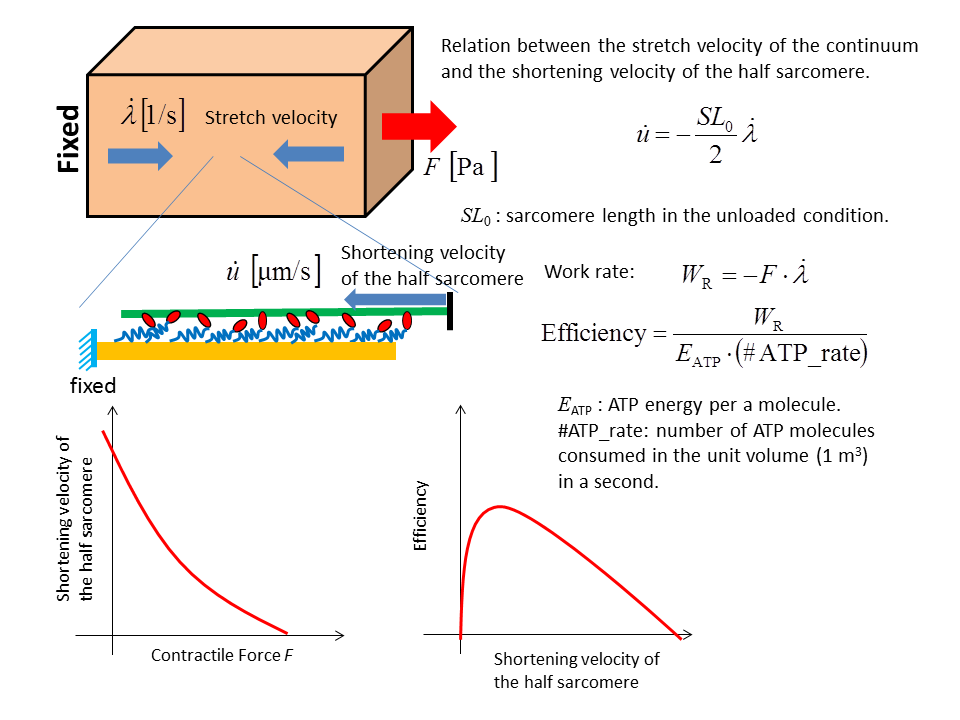# Observations

In this chapter, various observations provided by XB Modeler are introduced.

## Force Velocity Relationship

In this test, XB Modeler calculates the contractile force F per unit cross sectional area in the mechanical equilibrium condition for given shortening velocities. Here the sarcomere length is fixed with the natural length. The Monte Carlo simulation is performed for 0.25 seconds and the force is given by taking average in the second half duration [0.125,0.25]. You can see how the force F is computed in XB Modeler in Definition of contractile force. From the Simulation Results link, you can plot a force-velocity curve, an velocity-efficiency curve and an velocity-ATP consumption rate curve etc. The efficiency is calculated based on the ATP consumption rate.